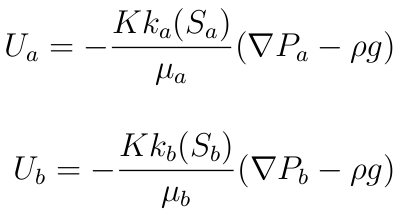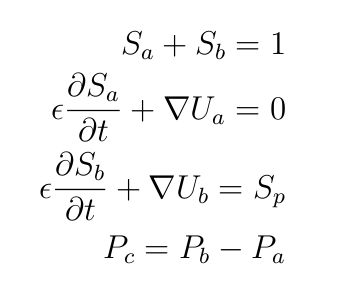# Models used

In the  developed solver we resolve the  conservation equations to obtain the saturation field and the pressure field.

In general case of an isothermal flow we have to calculate in each point of the porous media and as function of time :

• The pressure in each fluid $P_a$ and $P_{b}$
• The saturation in each fluid $S_a$ and $S_{b}$
• The velocity in each fluid $U_a$ and $U_{b}$

• The density in each fluid is $\rho_a$ and $\rho_{b}$, we supposed that the densities of fluids are equal and we call them  $\rho$ .
•  The viscosity in each fluid are $\mu_a$ and $\mu_{b}$

Then we have the following equations :

Darcy law :State equations :

We assume that the flow  is isothermal, moreover the density and the viscosity are supposed constant.

Continuity equations :